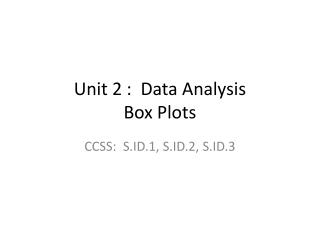DownloadDownload PresentationUnit 2 : Data Analysis Box Plots

# Unit 2 : Data Analysis Box Plots

Télécharger la présentation## Unit 2 : Data Analysis Box Plots

- - - - - - - - - - - - - - - - - - - - - - - - - - - E N D - - - - - - - - - - - - - - - - - - - - - - - - - - -
##### Presentation Transcript

1. Unit 2 : Data Analysis Box Plots CCSS: S.ID.1, S.ID.2, S.ID.3

2. Graphical Representations Recap • So far: Graphs are good because... • Dot plots are a good graphical representation of a distribution if ... • Other good graphical representations include box plots and histograms • Let’s discuss box plots now (histograms will be later)

3. Box plots look like...

4. What are the different parts that make up a box plot? • Minimum: • 1st Quartile: • Median: • 3rd Quartile: • Maximum:

5. Five-Number Summary • We use minimum, 1st quartile, median, 3rd quartile, and maximum to create box plots • Five numbers... We call this the five-number summary • Five number summary is always used to create box plots. Always.

6. Why do we use the median in box plots? • A median is a type of average • There are other types of averages, like a mean or a mode • What’s the difference between median, mean, mode? • Why do we use the median (and not the mean or mode) in box plots?

7. Let’s look at a simple distribution ... • To help us understand why we need to use the median in box plots • Cost of five randomly selected jeans were \$27, \$27, \$29, \$35, \$450 • What’s the mode? • What’s the mean? • What’s the median?

8. So we use the median when creating box plots because... • Discuss in your groups for one minute

9. We use the median in box plots because... • The median is resistant (median is not influenced by extreme values) • The median doesn’t change even if there is a value in the distribution that is very far away from all the other values • The median helps create a box plot that is not misleading

10. Let’s create a box plot. We asked a random sample of 21 juniors at Canyon how many songs they have on their iPods/mp3s.

11. Organize Data: • Arrange data smallest to greatest 2) Count in and find middle value (median) 3) Count in and find 1st quartile 4) Count in and find 3rd quartile 5) Identify min and max

12. Create box plot: Remember to put horizontal scale on bottom, labeled; label with values of each of the 5-number summary values; use context labels to clearly state what the box plot represents

13. SOCS (Shape, Outlier(s), Center, Spread) • Let’s analyze the data using SOCS

14. Student Heights from Last Class • Use the heights you put on the board last class to create a box plot. • Compare and contrast the dot plot and box plot (different graphical representations of the same data). Which is ‘better’??

15. What do you think are pros and cons of using a box plot?

16. Did you think of these possible advantages and disadvantages? • Pros/Advantages: • we see overall shape and trends well in box plot • we keep original data when doing a dot plot • Cons/Disadvantages: • we lose the original values when creating a box plot • sometimes more difficult to see trends and overall shape of data in dot plot if data is spread out a lot